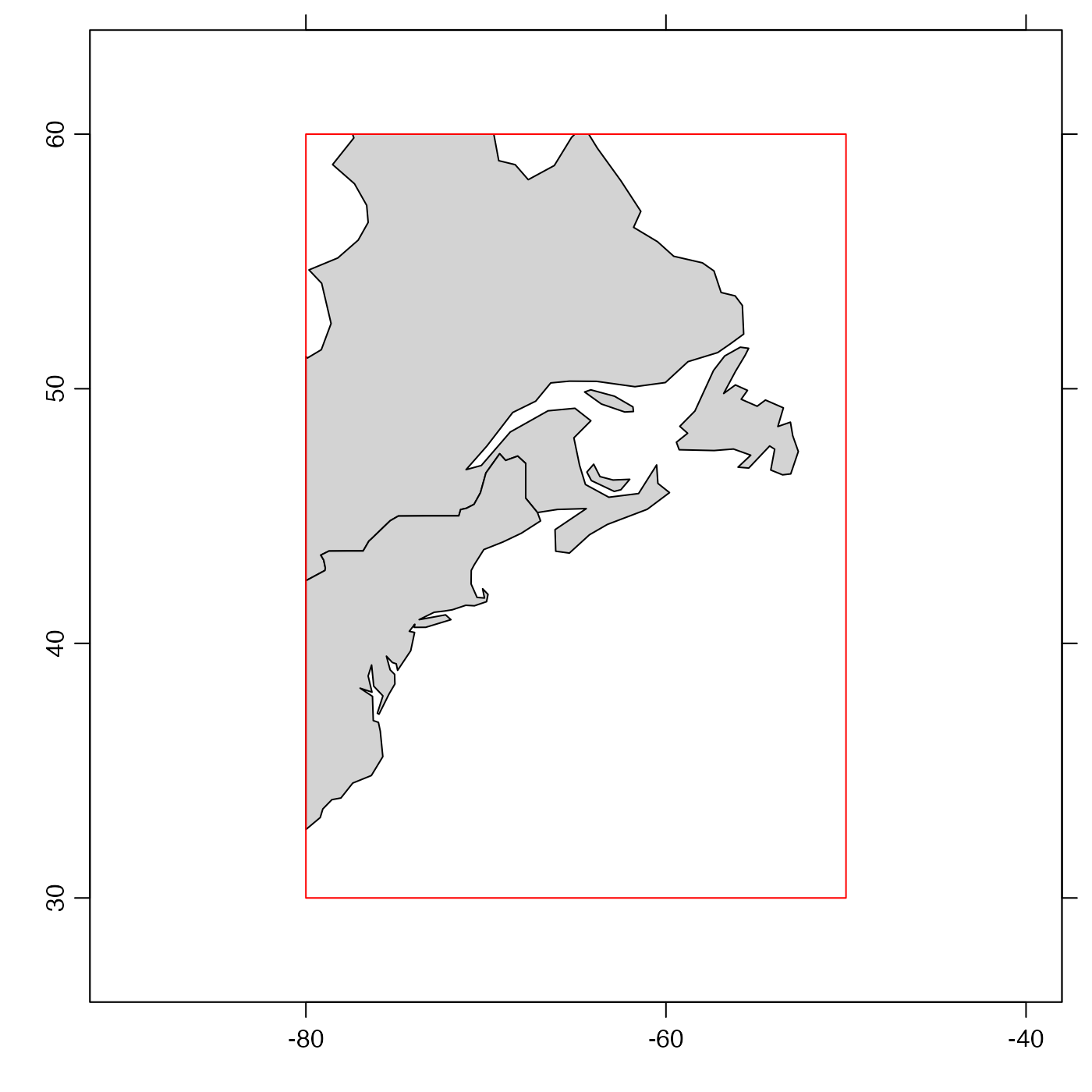Subsets a coastline object according to limiting values for longitude and latitude.

# S4 method for coastline
subset(x, subset, ...)

## Arguments

x

a coastline object.

subset

An expression indicating how to subset x. See “Details”.

...

optional additional arguments, the only one of which is considered is one named debug, an integer that controls the level of debugging. If this is not supplied, debug is assumed to be 0, meaning no debugging. If it is 1, the steps of determining the bounding box are shown. If it is 2 or larger, then additional processing steps are shown, including the extraction of every polygon involved in the final result.

## Value

A coastline object.

## Details

As illustrated in the “Examples”, subset must be an expression that indicates limits on latitude and longitude. The individual elements are provided in R notation, not mathematical notation (i.e. 30<latitude<60 would not work). Ampersands must be used to combine the limits. The simplest way to understand this is to copy the example directly, and then modify the stated limits. Note that > comparison is not permitted, and that < is converted to <= in the calculation. Similarly, && is converted to &. Spaces in the expression are ignored. For convenience, longitude and and latitude may be abbreviated as lon and lat, as in the “Examples”.

Other things related to coastline data: [[,coastline-method, [[<-,coastline-method, as.coastline(), coastline-class, coastlineBest(), coastlineCut(), coastlineWorld, download.coastline(), plot,coastline-method, read.coastline.openstreetmap(), read.coastline.shapefile(), summary,coastline-method

Other functions that subset oce objects: subset,adp-method, subset,adv-method, subset,amsr-method, subset,argo-method, subset,cm-method, subset,ctd-method, subset,echosounder-method, subset,lobo-method, subset,met-method, subset,oce-method, subset,odf-method, subset,rsk-method, subset,sealevel-method, subset,section-method, subset,topo-method, subset,xbt-method

Dan Kelley

## Examples

library(oce)
data(coastlineWorld)
# Subset to a box centred on Nova Scotia, Canada
if (requireNamespace("sf")) {
cl <- subset(coastlineWorld, -80<lon & lon<-50 & 30<lat & lat<60)
# The plot demonstrates that the trimming is as requested.
plot(cl, clon=-65, clat=45, span=6000)
rect(-80, 30, -50, 60, bg="transparent", border="red")
}